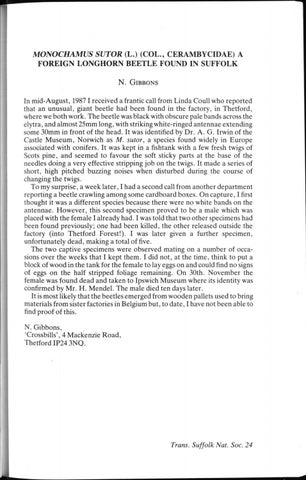MONOCHAMUS

SUTOR

(L.) (COL., C E R A M B Y C I D A E ) A

F O R E I G N L O N G H O R N B E E T L E F O U N D IN S U F F O L K N . GIBBONS

In m i d - A u g u s t , 1987 I received a frantic call f r o m Linda Coull w h o r e p o r t e d that an u n u s u a l , giant beetle had b e e n f o u n d in t h e factory, in T h e t f o r d , where we b o t h w o r k . T h e beetle was black with obscure pale b a n d s across the elytra, and almost 2 5 m m long, with striking white-ringed a n t e n n a e extending some 3 0 m m in f r o n t of t h e h e a d . It was identified by D r . A . G . Irwin of the Castle M u s e u m , Norwich as M. sutor, a species f o u n d widely in E u r o p e associated with conifers. It was kept in a fishtank with a few fresh twigs of Scots p i n e , a n d s e e m e d to f a v o u r t h e soft sticky parts at the base of the needles d o i n g a very effective stripping j o b on t h e twigs. It m a d e a series of short, high pitched buzzing noises w h e n disturbed during the course of changing t h e twigs. T o my s u r p r i s e , a w e e k later, I had a second call f r o m a n o t h e r d e p a r t m e n t reporting a beetle crawling a m o n g s o m e c a r d b o a r d boxes. O n c a p t u r e , I first thought it was a d i f f e r e n t species because t h e r e were n o white b a n d s on the a n t e n n a e . H o w e v e r , this s e c o n d specimen proved to b e a male which was placed with t h e f e m a l e I already had. I was told that t w o o t h e r specimens had been f o u n d previously; one had been killed, the o t h e r released outside the factory (into T h e t f o r d Forest!). I was later given a f u r t h e r s p e c i m e n , u n f o r t u n a t e l y d e a d , m a k i n g a total of five. T h e t w o captive specimens were observed mating on a n u m b e r of occasions over t h e w e e k s that I kept t h e m . I did n o t , at the time, think to put a block of w o o d in the t a n k for the f e m a l e to lay eggs on and could find no signs of eggs on t h e half stripped foliage remaining. O n 30th. N o v e m b e r the female was f o u n d d e a d and t a k e n to Ipswich M u s e u m w h e r e its identity was confirmed by M r . H . M e n d e l . T h e male died ten days later. It is m o s t likely that t h e beetles e m e r g e d f r o m w o o d e n pallets used to bring materials f r o m sister factories in Belgium but, to d a t e , I have not b e e n able to find proof of this. N. G i b b o n s , 'Crossbills', 4 M a c k e n z i e R o a d , T h e t f o r d IP24 3 N Q .

Trans. Suffolk

Nat. Soc. 24

Gibbons, N.

Gibbons, N.